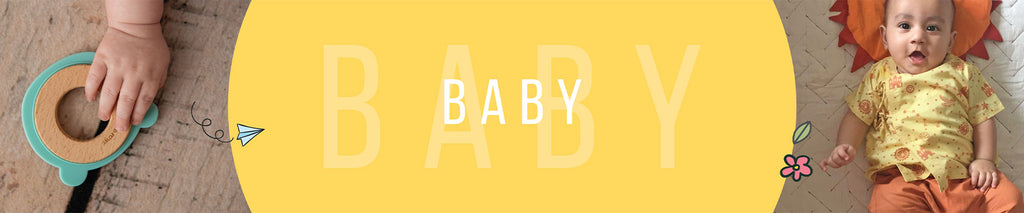Free Shipping PAN INDIARs. 499.00

Rs. 499.00

Rs. 499.00

Rs. 799.00

Rs. 499.00

Rs. 499.00

Rs. 499.00

Rs. 599.00

Rs. 599.00

Rs. 369.00

Rs. 699.00

Rs. 369.00

Rs. 699.00

Rs. 699.00

Rs. 699.00

Rs. 699.00

Rs. 699.00

Rs. 699.00

Rs. 699.00

Rs. 699.00

Rs. 699.00

Rs. 699.00

Rs. 699.00

Rs. 699.00

Rs. 699.00

Rs. 699.00

Rs. 499.00

Rs. 499.00

Rs. 499.00

Rs. 399.00

Rs. 349.00

Rs. 669.00

Rs. 669.00

Rs. 669.00

Rs. 669.00

Rs. 1,099.00